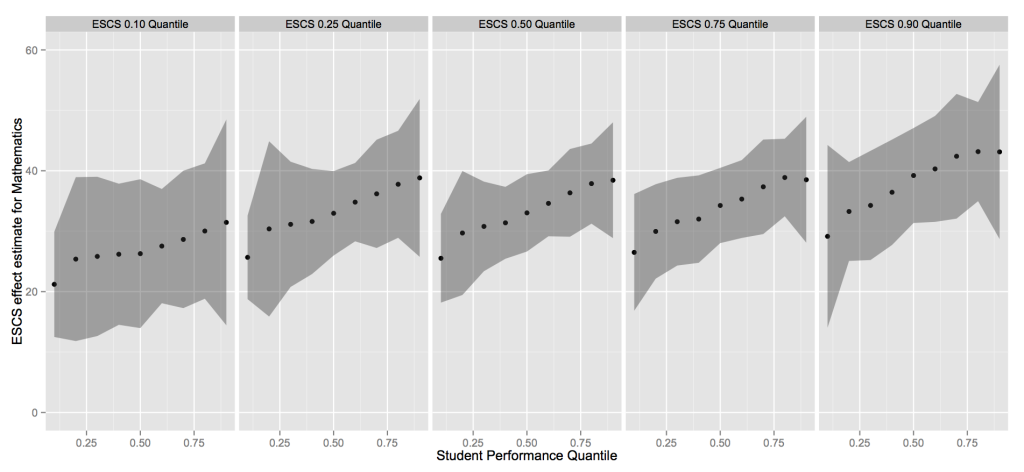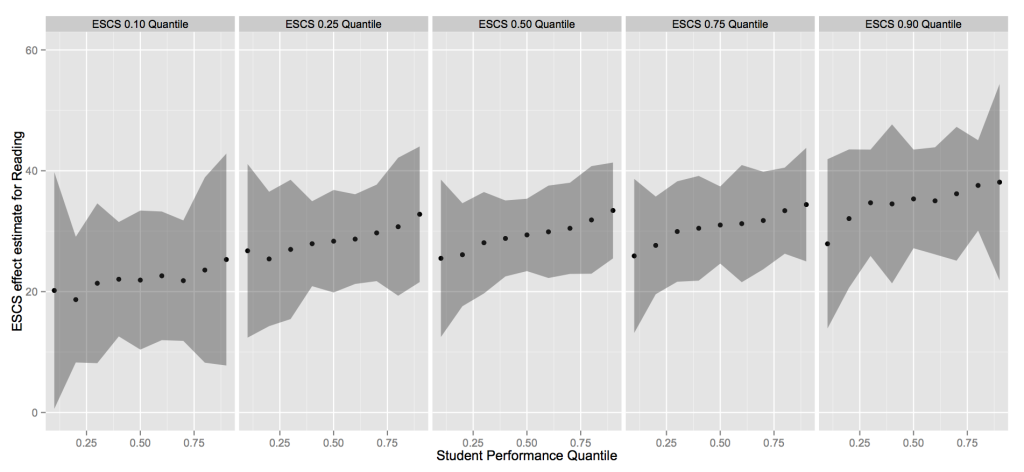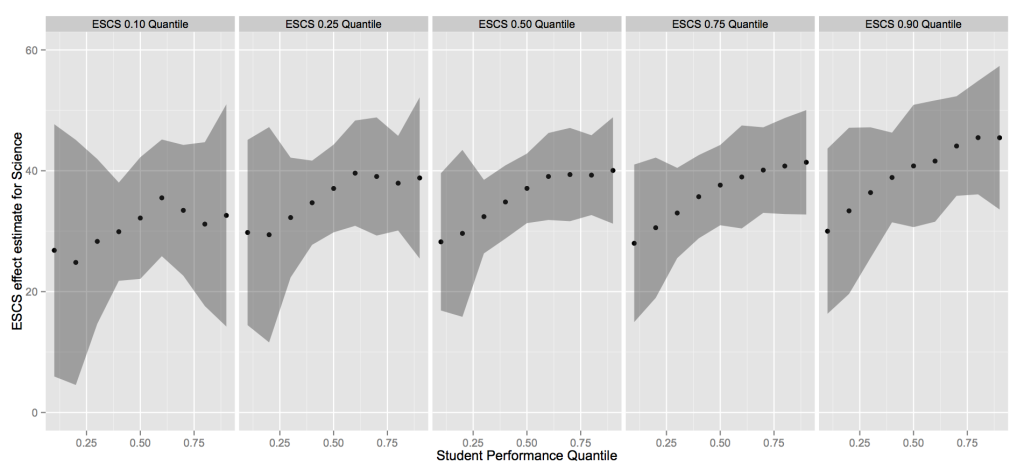# PISA 2012 – Economic, Social, and Cultural Status

It has been known for a while that academic performance is positively correlated with economic, social, and cultural status (ESCS). The causal factors that influence inequality in educational outcome are likely to be byproducts or sources of the variation in ESCS.

Using the PISA 2012 survey results for only the USA, the influence of ESCS on student performance in mathematics, science, and reading, was modeling using a quantile regression approach for recursive structural equations (Ma and Koenker, 2006). The model was constructed with ESCS as the structural effect of interest. Home educational $(x_1)$ resources, cultural possessions $(x_2)$, wealth $(x_3)$, and parent’s education $(x_4)$ were used to model ESCS (Y). This effect was then introduced into the a model of student scores (y). The explicit model follows,

$Y_i = \alpha_0+\alpha_1x_{i1} + \alpha_2x_{i2} + \alpha_3x_{i3} + \alpha_4x_{i4} + \nu_{i2}$
$y_i = \beta_0 + \beta_1Y_i + \beta_2x_{i1} + \beta_3x_{i2} + \beta_4 x_{i3} + \nu_{i1} + \lambda\nu_{i2}$

where i=1,…,10,294 and $\beta_1$ represents the structural effect of ESCS evaluated at several different quantile combinations. Five quantiles of ESCS were considered (0.1,0.25,0.5,0.75,0.9) and 9 quantiles of the student achievement (0.1,0.2,0.3,0.4,0.5,0.6,0.7,0.8,0.9). Each of the plots below show the estimated value for the $\beta_1$. As we can see, the estimated effects for the achievement quantiles are very similar in the middle quantiles of ESCS (0.25,0.50,0.75). In the tail quantiles of ESCS (0.1,0.9), there are some interesting features. For the largest for 0.9 quantile of ESCS, the estimated effect are the largest. There is also a larger range between the highest and lowest estimated effects for the 0.9 quantile of ESCS than in the smaller quantiles of ESCS.Estimated effects of ESCS on math scores at several quantiles of both ESCS and math achievementEstimated effects of ESCS on reading scores at several quantiles of both ESCS and reading achievementEstimated effects of ESCS on science scores at several quantiles of both ESCS and science achievement

Even after accounting for several potential influences like a parent’s educational achievement, wealth, home possessions, and educational resources, the effect of ESCS on student outcomes is still very significant. Our approach to evaluating inequality in these outcomes breaks down this effect by quantiles and allows policy makers to assess the degree of inequality within and among the highest and lowest achieving students. Given the potential economic benefits, it is imperative that policy makers find a way to improve the performance of our lowest achieving students. In order for policy makers to provide an effective course of action, it is necessary that they are able to assess the role of ESCS on inequality in educational outcome along with having options that can be more easily manipulated than ESCS.